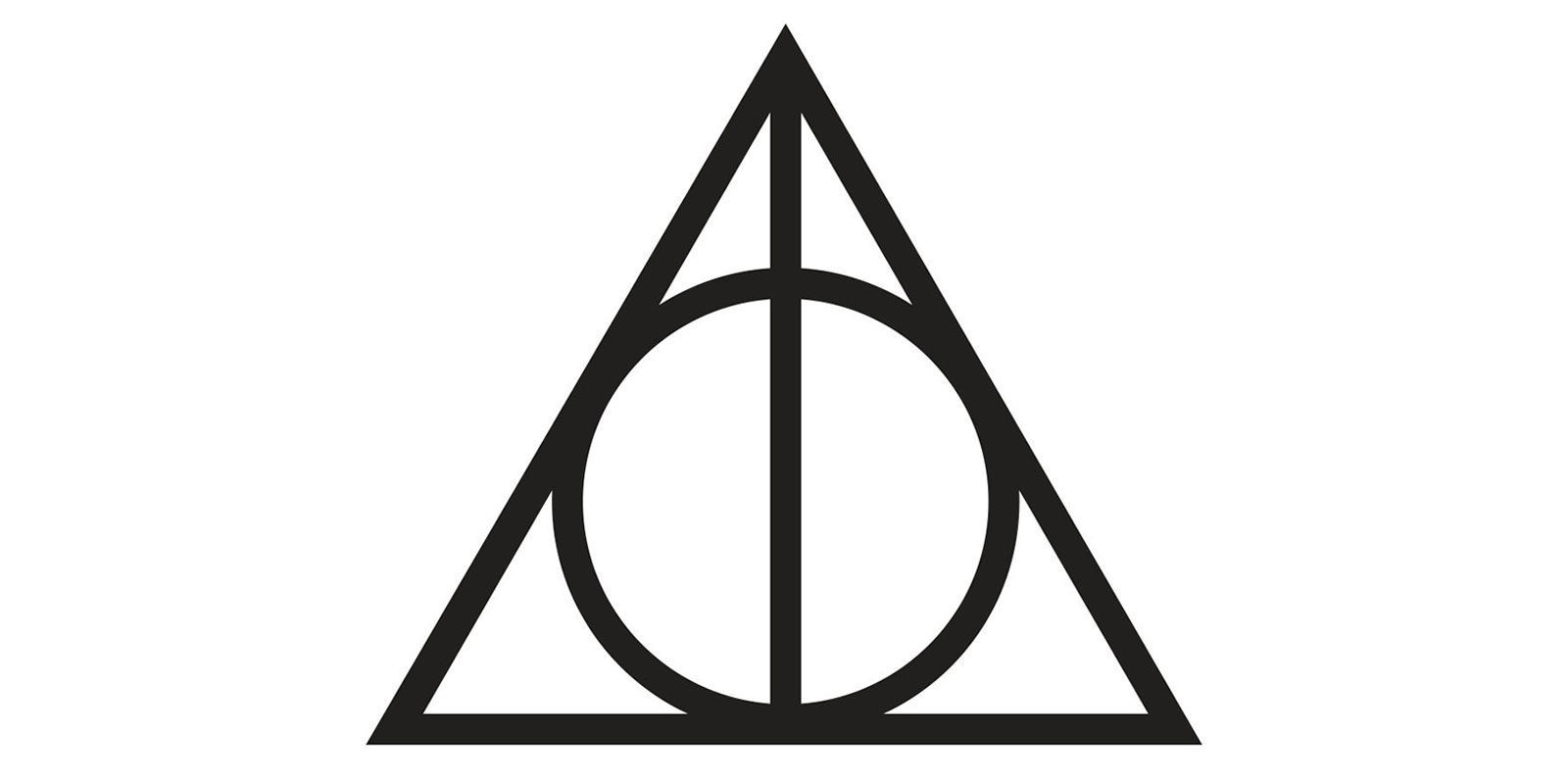# How to deal with Tim

My friend Tim is a real dick. Whatever happens to him he just won't die! I asked him what the secret to his power was (So I could kick his ass). He handed me a crumpled up piece of this paper with some numbers on it, gave me a wedgie and walked off.

The numbers are:

1 , 10 , 6 , 3 , 15
1 , 4 , 5 , 1 , 3
3 , 1.5 , 0.5 , 7.5 , 5

What can I do (If anything) to beat him?

Hint 1:

These are 3 individual sets of numbers that may not be in order

Hint 2:

Each sequence gives a different part of the answer

Hint 3:

Two of the sequences are similar

Hint 4:

An optional sequence to also consider is 2, 0.5, 0.5, 1.5, 2.5

• I have already bookmarked this question. If someone answer to this question, will I get a notification? May 14, 2018 at 11:22
• If you got one now, than yes :) May 14, 2018 at 12:49
• @BálintBudavölgyi Unfortunately no :-( I just came to site to check. No notification... May 16, 2018 at 4:23
• @IamtheMostStupidPerson tagging a '@username' like this as the first part of a comment will notify that user. Also, some partial answers have been provided. May 16, 2018 at 8:17
• Anything to do with rot13(uggc://jjj.qvtvgnyfcl.pbz/zbivrf/uneel-cbggre/arjf/n841654/frperg-vafcvengvba-uneel-cbggre-qrnguyl-unyybjf-flzoby/)? May 17, 2018 at 12:58

Going off @Bálint Budavölgyi's comment, I think the answer might be:

The Deathly Hallows, from Harry Potter

My reasoning is:

As Hint 1 says, the numbers in each list are not in order, so we must reorder them first.
The first line can be reordered as 1, 3, 6, 10, 15...; this has already been solved and verified as the start of the sequence of triangular numbers.
The second line can be reordered as 3, 1, 4, 1, 5...; this has already been solved and verified as the first digits of pi, the circle constant.
The third line (now updated) can be reordered as 0.5, 1.5, 3, 5, 7.5...; this sequence is the same as the first, but halved. (1/2, 3/2, 6/2, 10/2, 15/2...) This is why Hint 3 suggests that two of the sequences are similar.
Hint 4 suggests that the third sequence could also be 2, 0.5, 0.5, 1.5, 2.5. This can be reordered as 1.5, 0.5, 2, 0.5, 2.5...;, which is the same as the second sequence but halved.

These sequences clue towards:

the triangle, the circle, and then dividing one or both of those shapes in half.
Combining and overlapping these images gives the symbol for the Deathly Hallows, a collection of powerful artifacts in the Harry Potter universe said to have been created by Death.
"According to legend, he who possesses these three artefacts would become the Master of Death," which would explain why we can't kill Tim.
(In reference to Hint 2, the three aspects of the image each represent one of the artefacts. Specifically, the triangle represents the Cloak of Invisibility; the circle represents the Resurrection Stone; and the line that divides the image in half represents the Elder Wand.)• Seems like a good answer, but I would expect a "trivia" tag if this were the case. You also needed to modify one of the sequences. May 17, 2018 at 14:09
• Ahh I totally missed that 7.5 apologies. You've got the symbol right, can you elaborate on the other hints/parts of the riddle May 17, 2018 at 14:27
• Updated my answer to elaborate on the hints. May 17, 2018 at 14:59

the first line is:

Triangular Number Sequence -> 1, 3, 6, 10, 15, 21, 28, 36, 45, ... (https://www.mathsisfun.com/algebra/triangular-numbers.html)

• Yep you've solved this line May 16, 2018 at 8:17

The second line is

9, because the numbers can be rearranged to get $3, 1, 4, 1, 5, \_$, which are the first digits of $\pi$.

• Yep you've solved that line. The answer is pi though not 9 May 15, 2018 at 15:15
• Why did you say 9? May 16, 2018 at 9:14
• Its the next number in pi : 3.14159 May 16, 2018 at 10:30
• Got me thinking the answer could be something like 'happiness', but I can't really see a link between first sequence and 'hap', or the last sequence and 'ness'.. We may however be looking for letters rather then nummers. May 16, 2018 at 12:25
• @TimCouwelier It could also shapes. The first line is triangles, the second line is the circle constant.
– user27014
May 16, 2018 at 13:20

The first line could be:

$21$, because

If you sort the numbers ascending, you get $1, 3, 6, 10, 15$.
Then if you subsctract the previous number from the actual, add it to the actual, and then add +1 you get the next number.

$x_n = x_{n-1} + (x_{n-1} - x_{n-2}) + 1$

$15 + (15 - 10) + 1 = 21$

It is definitely a stretch, but the second line could be:

$8$, as

You sort the numbers ascending again

$1, 1, 3, 4, 5$

$x_n = x_{n-1} + x_{n-3}$

$5 + 3 = 8$

And I have a feeling that

If you add up the answers of the three individual rows, you will get: 42

• Am I on the right path? May 15, 2018 at 12:47
• Not for 42, having the first line being ascending is correct though May 15, 2018 at 12:52